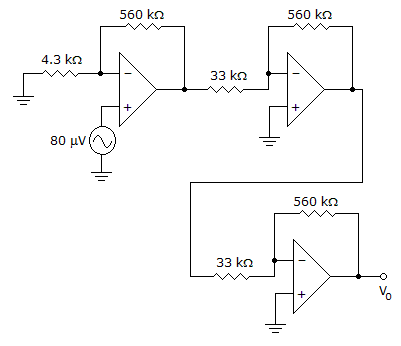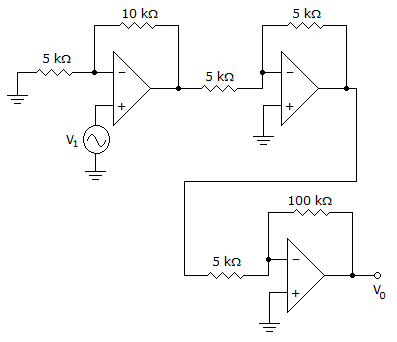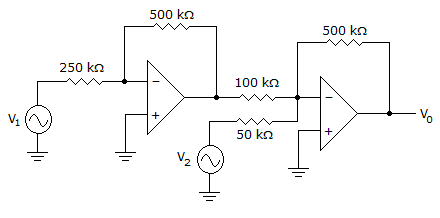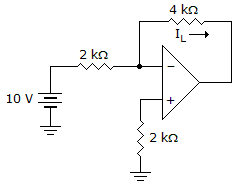# Electronic Devices - Op-Amp Applications

### Exercise :: Op-Amp Applications - General Questions

21.

Calculate the output voltage.A. 3.02 V B. 2.03 V C. 1.78 V D. 1.50 V

Explanation:

No answer description available for this question. Let us discuss.

22.

Calculate the output of the second stage op-amp if V1 = 25 mV.A. –0.075 V B. 0.525 V C. 0.06 V D. 4.2 V

Explanation:

No answer description available for this question. Let us discuss.

23.

A difference between a passive filter and an active filter is that a passive filter uses amplifier(s), but an active filter does not.

 A. True B. False

Explanation:

No answer description available for this question. Let us discuss.

24.

Calculate the output voltage Vo if V1 = –V2 = 300 mV.A. 0 V B. –6 V C. 6 V D. –8 V

Explanation:

No answer description available for this question. Let us discuss.

25.

Calculate IL for this circuit.A. 3 mA B. 4 mA C. 5 mA D. 6 mA